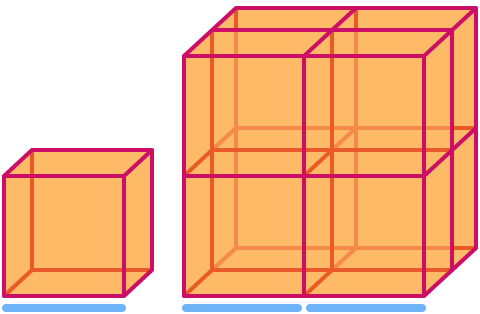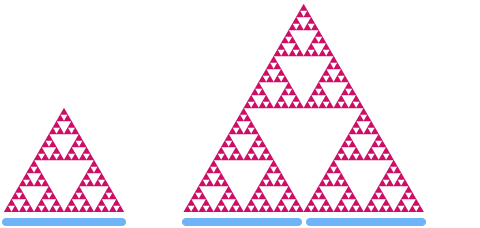## 용어 사전

왼쪽에있는 키워드 중 하나를 선택하세요…

# FractalsIntroduction

독서 시간: ~45 min

When looking around nature, you might have noticed intricate plants like these:This Fern consists of many small leaves that branch off a larger one.This Romanesco broccoli consists of smaller spiralling around a larger one.

Initially, these appear like highly complex shapes – but when you look closer, you might notice that they both follow a relatively simple pattern: all the individual parts of the plants look exactly the same as the entire plant, just smaller. The same pattern is repeated over and over again, at smaller scales.

In mathematics, we call this property self-similarity, and shapes that have it are called fractals. They are some of the most beautiful and most bizarre objects in all of mathematics.

To create our own fractals, we have to start with a simple pattern and then repeat it over and over again, at smaller scales.

One of the simplest patterns might be a line segment, with two more segments branching off one end. If we repeat this pattern, both of these blue segments will also have two more branches at their ends.

You can move the blue dots to change the length and angle of all of the branches. Then increase the number of iterations using the slider below.

Depending on the position of the branches, you can make completely different patterns – looking like the above, a , or . What else can you find?

Another famous fractal is the Sierpinski triangle. In this case, we start with a large, equilateral triangle, and then repeatedly cut smaller triangles out of the remaining parts.

Notice how the final shape is made up of three identical copies of itself, and each of these is made up of even smaller copies of the entire triangle! You could keep zooming into the triangle forever, and the patterns and shapes will always continue repeating.

The plants at the beginning of this chapter look just like fractals, but it is clearly impossible to create true fractals in real-life. If we keep repeating the same pattern over and over again, smaller and smaller, we would eventually get to cells, molecules or atoms which can no longer be divided.

However, using mathematics, we can think about the properties that real fractals “would” have – and these are very surprising…

## Fractal DimensionsFirst, let’s think about the dimension of fractals. A line has dimension . When scaling it by a factor of 2, its length increases by a factor of 21=2. Obviously!A square has dimension . When scaling it by a factor of 2, its area increases by a factor of 22= .A cube has dimension . When scaling it by a factor of 2, its volume increases by a factor of 23= . Notice that the larger cube in the image consists of 8 copies of the smaller one!Now let’s have a look at the Sierpinski triangle. If we scale it by a factor of 2, you can see that it’s “area” increases by a factor of .

Let’s say that d is the dimension of the Sierpinski triangle. Using the same pattern as above, we get 2d=3. In other words, d = ≈ 1.585…

But wait … how can something have a dimension that is not an integer? It seems impossible, but this is just one of the weird properties of fractals. In fact, this is what gives fractals their name: they have a fractional dimension.

With every iteration, we remove some of the area of the Sierpinski triangle. If we could do this infinitely many times, there would actually be no area left: that’s why the Sierpinski triangle is something in-between a two-dimensional area, and a one-dimensional line.

While many fractals are self-similar, a better definition is that fractals are shapes which have a non-integer dimension.

## The Koch Snowflake

There are many shapes in nature that look like fractals. We’ve already seen some plants at the beginning of this chapter. Other great examples are snowflakes and ice crystals:

To create our own fractal snowflake, we once again have to find a simple procedure we can apply over and over again.

Like the Sierpinski triangle, let’s start with a single, equilateral triangle. However, rather than removing smaller triangles at every step, we add smaller triangles along the edge. The side-length of every triangle is of the triangles in the previous step.

The resulting shape is called the Koch snowflake, named after the Swedish mathematician Helge von Koch. Notice, once again, that small sections of the edge of the snowflake look exactly the same as larger sections.When we scale one edge segment of the Koch Snowflake by a factor of 3, its length .

Using the same relationship between dimensions and scale factors as above, we get the equation . This means that the dimension of the Koch Snowflake is d=log341.262.

### Area

Creating the Koch snowflakes is almost like a recursive sequence: we know the starting shape (a triangle), and we know how to get from one term to the next (by adding more triangles on every edge):new trianglesnew trianglesnew triangles

After the first iteration, the number of new triangles added increases by a factor of at every step. At the same time, the area of these new triangles decreases by a factor of at every step.

Let’s say that the first triangle has an area of 1. Then the total area of the next three triangles is 3×19=13. The following steps all form a , with common ratio .

Using the formula for the sum of infinite geometric series, we can calculate that the total area of the Koch snowflake is

A=1+13×1=85=1.6.

### Perimeter

We can also try to calculate the perimeter of the Koch snowflake. As we have already seen before, the length of the perimeter changes by a factor of at every step.

This means that, once again, we have a geometric series – but in this case, it . This means that the perimeter of the Koch snowflake is actually infinitely long!If this seems counterintuitive, just remember that we multiply the perimeter by 43 at every step, and we do this infinitely many times.

It is almost unthinkable that you can have a shape with a finite area and also an infinite circumference – but this is just one of the many unexpected properties of fractals.

Can you come up with any other ways to create your own fractals?

“My soul is spiralling on frozen fractals all around…”

## Menger Sponge

Fractals don’t have to be “flat”, like many of the examples above. One of the most famous fractals that look three-dimensional is the Menger sponge, named after the mathematician Karl Menger who first described it in 1926.

We start with a solid cube, and repeatedly drill smaller and smaller holes into its sides. Every new iteration of holes has the width of the previous iteration of holes.

A 3×3×3 cube consists of 27 smaller cubes, but here we have removed some of these. The Menger sponge consists of copies of itself, which are 3 times smaller.

Now we can try to calculate the dimension d of the Menger sponge just like we did for the Koch snowflake above. In this case we get 3d=20, or d=log3202.727.

If you imagine cutting out more and more holes, infinitely many times, there would be no actual volume left. That’s why the cube is “not quite” three-dimensional!

## Fractal Coastlines

One of the key characteristics of all the fractals we’ve seen so far is that you can “zoom in” forever and always find new patterns. Around 1920, the British mathematician Lewis Fry Richardson realised that the same is true for the border or coastline of many countries.

You start with the basic shape of the country, and, as you zoom in, you add river inlets, bays and estuaries, then individual cliffs, rocks, pebbles, and so on:

This is a significant problem when trying to calculate the length of the border of a country – how do you decide how far to zoom in, and which nooks and crannies to include?

One way we could measure the length of Britain’s coastline, for example, is to take a long ruler, walk all the way around its beaches, and then add up all the distances.

If the ruler is \${rulers[index]}km long, we have to use it \${count} times, so we get a total coastline of \${count} × \${rulers[index]} = \${count * rulers[index]}km.

We can just keep going, with smaller and smaller rulers, and each time our result for the length of the coastline would get a bit longer. Just like the Koch Snowflake before, it seems that Britain’s coastline is infinitely long! This is often called the coastline paradox.

A few decades later, the mathematician Benoit Mandelbrot stumbled upon Richardson’s work in a discarded library book, while working at IBM. He recognised its significance, and also how it relates to more recent research on fractals and dimensions.

The coastline of Britain certainly “looks” fractal, but it is not self-similar, like other fractals we’ve seen before. In order to find its size, we can draw it on a grid and count the number of cells that it intersects with.

Initially, there are 88 intersecting cells. If we scale the coastline by a factor of 2, there are 197 intersecting cells – more than twice as many!

The size of the coastline has increased by a factor of 19788. Like before, this means that the dimension of the coastline is

d=log2197881.16

If we repeat this with larger grids, we’d find that the dimension of Britain’s coastline is actually approximately 1.21. Mandelbrot realised that this fractal dimension is also a measure of the roughness of a shape – a new concept, for which he found important applications in many other areas of mathematics and science.

## More Fractals in Nature and Technology

While true fractals can never appear in nature, there are many objects that look almost like fractals. We’ve already seen plants, snowflakes and coastlines, and here are some more examples:

Mountain range in central Asia

Ganges river delta in India

Lightning bolts

Blood vessels in the retina

Grand Canyon in the USA

Clouds

All these objects might appear completely random, but, just like fractals, there is an underlying pattern that determines how they are formed. Mathematics can help us understand the shapes better, and fractals have applications in fields like medicine, biology, geology and meteorology.

Computer-generated fractal terrain

We can also use fractals to create realistic “copies” of nature, for example, as landscapes and textures used in video games or computer-generated movies. The water, mountains and clouds in this image are made entirely by a computer, with the help of fractals!

And we can even reverse this process to compress digital images, to reduce their file size. The first algorithms were developed by Michael Barnsley and Alan Sloan in the 1980s, and new ones are still being researched today.

Archie